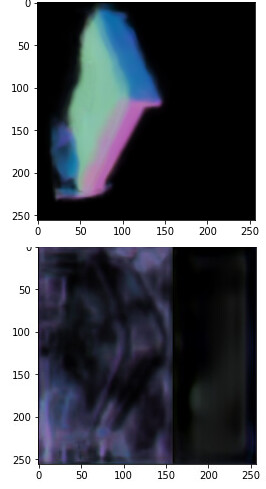``````import torch

device = torch.device("cuda:0" if torch.cuda.is_available() else "cpu")
device
``````
``````normal = ResNetUNet(3).to(device)
depth = ResNetUNet(1).to(device)
``````
``````normal_save_name = 'BKP_module1_normal_full_32_epochs.pt'
path_to_normal = F"/content/gdrive/My Drive/{normal_save_name}"
``````
``````depth_save_name = 'BKP_module1_depth_full_10_epochs.pt'
path_to_depth = F"/content/gdrive/My Drive/{depth_save_name}"
``````
``````mask_save_name = 'BKP_module1_mask_full_11_epochs.pt'
``````
``````normal.load_state_dict(normal_dict)

normal.eval()
depth.eval()
``````

And then I use this code to forward and plot the result of first network:

``````data = json.load(open('pix3d.json'))

batch = transforms.ToTensor()(batch)
batch = torch.unsqueeze(batch, 0)
batch = batch.to(device)

normal_map = normal(batch)
normal_map = normal_map.cpu().detach().numpy()

plt.imshow(normal_map.T)
``````

So if I load only the first network the result looks pretty okay for my purposes. But if I use the code above to load all of my three networks I get garbage results:Above are results of running the same code but with 1 and 3 networks loaded respectively.
My first thought was that the problem is with weights. But I checked that state dict of first network is the same both times. So I have literally no I idea why this is happening.
Any help will be much appreciated.